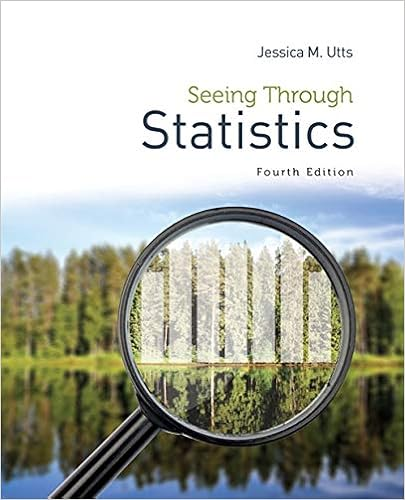# 6 2 3 4 2 5 1 n n n n 6n 23n 4n 25n1 6n 2 3n 4n 10n 2

• Test Prep
• 9
• 83% (12) 10 out of 12 people found this document helpful

This preview shows page 4 - 8 out of 9 pages.

##### We have textbook solutions for you!
The document you are viewing contains questions related to this textbook.The document you are viewing contains questions related to this textbook.
Chapter 16 / Exercise 11
Seeing Through Statistics
UttsExpert Verified
6 2 3 4 2 5 1 n n n n   6n-(2+3n) = -4n-2(5n+1) 6n-2-3n = -4n-10n-2 6n – 3n – 2 = -14n – 2 3n – 2 = -14n – 2 3n – 3n – 2 = -14n – 3n – 2 -2 = -17n – 2 -2 + 2 = -17n – 2 + 2 0 = -17n 0 17 = 17 n 17 0 = n
4) Solve the equation below. Show all work following the methods discussed in class; if an equation includes fractions, clear the fractions in the first step. If the equation has a unique solution, please show the complete check of your answer.
##### We have textbook solutions for you!
The document you are viewing contains questions related to this textbook.The document you are viewing contains questions related to this textbook.
Chapter 16 / Exercise 11
Seeing Through Statistics
UttsExpert Verified
Math 012 Quiz 1 Page 5 CHECK. 3 2 ( 1 )− 8 3 = 1 6 ( 1 )− 4 3 ( 1 ) 3 2 ( 3 3 )− 8 3 ( 2 2 )= 1 6 ( 1 1 ) 4 3 ( 2 2 ) 9 6 16 6 = 1 6 8 6 7 6 = 7 6 5) Solve the equation below. Show all work following the methods discussed in class; if an equation includes fractions, clear the fractions in the first step. If the equation has a unique solution, please show the complete check of your answer. 2 8
Math 012 Quiz 1 Page 6 9 8 = 9 8 6) The number of kilograms of water in a person’s body varies directly as the person’s mass. person with a mass of 90 kg contains 60 kg of water. How many kilograms of water are in a person with a mass of 54 kg? A
D. There are 36 kilograms of water in a person with a mass of 54 kilograms.
Math 012 Quiz 1 Page 7 7) In kick boxing, the energy needed to break a board of standard width and thickness
•••